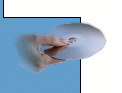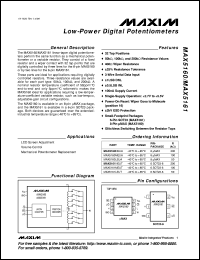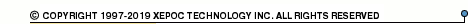More than478 253 registered clientsMAX5175 series datasheets. Manufacturer: Maxim Integrated Products.

 MAX5175AEEE Low-power, serial, 12-bit DAC with force/sense voltage output. Single-supply +5V operation. Full-scale output range +2.048V(Vref=+1.25V). INL(LSB) +-1. in 16-pin QSOP package. Operational temperature range from -40°C to 85°C. Datasheet*) MAX5175BEEE Low-power, serial, 12-bit DAC with force/sense voltage output. Single-supply +5V operation. Full-scale output range +2.048V(Vref=+1.25V). INL(LSB) +-2. in 16-pin QSOP package. Operational temperature range from -40°C to 85°C. Datasheet*) MAX5177AEEE Low-power, serial, 12-bit DAC with force/sense voltage output. Single-supply +3V operation. Full-scale output range +4.096V(Vref=+2.5V). INL(LSB) +-2. in 16-pin QSOP package. Operational temperature range from -40°C to 85°C. Datasheet*) MAX5177BEEE Low-power, serial, 12-bit DAC with force/sense voltage output. Single-supply +3V operation. Full-scale output range +4.096V(Vref=+2.5V). INL(LSB) +-4. in 16-pin QSOP package. Operational temperature range from -40°C to 85°C. Datasheet*) MAX5175 Low-Power, Serial, 12-Bit DACs with Force/Sense Voltage Output Datasheet*) MAX5175AEEE+ Low-Power, Serial, 12-Bit DACs with Force-Sense Voltage-Output. Lead-free Datasheet*) MAX5175AEEE+T Low-Power, Serial, 12-Bit DACs with Force-Sense Voltage-Output. Lead-free Datasheet*) MAX5175AEEE-T Low-Power, Serial, 12-Bit DACs with Force-Sense Voltage-Output Datasheet*) MAX5175BEEE+ Low-Power, Serial, 12-Bit DACs with Force-Sense Voltage-Output. Lead-free Datasheet*) MAX5175BEEE+T Low-Power, Serial, 12-Bit DACs with Force-Sense Voltage-Output. Lead-free Datasheet*) MAX5175BEEE-T Low-Power, Serial, 12-Bit DACs with Force-Sense Voltage-Output Datasheet*) MAX5177AEEE+ Low-Power, Serial, 12-Bit DACs with Force-Sense Voltage-Output. Lead-free Datasheet*) MAX5177AEEE+T Low-Power, Serial, 12-Bit DACs with Force-Sense Voltage-Output. Lead-free Datasheet*) MAX5177AEEE-T Low-Power, Serial, 12-Bit DACs with Force-Sense Voltage-Output Datasheet*) MAX5177BEEE+ Low-Power, Serial, 12-Bit DACs with Force-Sense Voltage-Output. Lead-free Datasheet*) MAX5177BEEE+T Low-Power, Serial, 12-Bit DACs with Force-Sense Voltage-Output. Lead-free Datasheet*) MAX5177BEEE-T Low-Power, Serial, 12-Bit DACs with Force-Sense Voltage-Output Datasheet*)